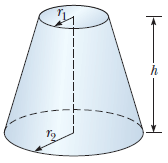# Problem: The figure shows a frustrum of a cone. Match each of the expressions (a) π(r1 + r2)[h2 + (r2 − r1)2]1/2 (b) 2π(r1 + r2) and (c) πh(r12 + r1r2 + r22)/3 with the quantity it describes: (1) the total circumference of the flat circular faces (2) the volume or (3) the area of the curved surface

###### FREE Expert Solution

In this problem, we'll verify the correct expression against the proper dimension of the quantity described.

We'll assume the lengths are in meters.

Circumference dimension: L (m)

91% (471 ratings)###### Problem Details

The figure shows a frustrum of a cone. Match each of the expressions (a) π(r1 + r2)[h2 + (r2 − r1)2]1/2 (b) 2π(r1 + r2) and (c) πh(r12 + r1r2 + r22)/3 with the quantity it describes: (1) the total circumference of the flat circular faces (2) the volume or (3) the area of the curved surface